## Is there a ceiling function like in excel?

### Is there a ceiling function like in excel?

Hi,
Like the title says... I'm looking for a way to replicate the ceiling function in excel. I played around with ceil but either I'm not getting it or it doesn't do the same as excel.

I basically want to round off numbers/measurments to the nearest 1/64.

Any help would be greatly appreciated.

Frank
0

Frankn

### Re: Is there a ceiling function like in excel?

If n is your number, then multiplying it by 100 and rounding it would
round it to 2 decimal places, then divide it by 100 to get your decimal
back...
n = 12.3456
n * 100 = 1234.56
round(n) = 1235
1235 / 100 = 12.35

So to make a simple flexible function [method] use
def round_to(n, x)
(n*10**x).round.to_f/10**x
end

Usage: where n=12.3456 and you want 2dps...
n=round_to(n, 2)
>>> 12.35
If you are then turning the value into a string you can use this one step
strn=sprintf("%.2f", n)
>>> "12.35"
Again adjust the numerical part to suit the dp's desired...
0
TIGTIG
Global Moderator

### Re: Is there a ceiling function like in excel?

Thank you Tig... that is helpful but it doesn't accomplish exactly what I'd like it to do... unless I'm not using it properly.

Here's a more concrete example of what my problem is...

A result I get after doing some funky math is 0.17578125 but I'd like to round that off to 0.1875. In Excel I'd this =CEILING(0.17578125, 1/64) and that works great.

0,17578125 is actually more accurate but it's TOO accurate.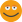Because I get a measurement inside Sketchup that has the ~ in front of it.

Basically I'de likt to set the accuracy of my formulas to 1/64.

I appologize if it's me not 'getting' your previous answer... I guess from my question you already guessed I'm still new to this whole programming deal.0

Frankn

### Re: Is there a ceiling function like in excel?

I'm pretty sure nothing like that is built into ruby. At least not the 1.8 branch of ruby.

Here is my hacked together version. I would guess others here might have a cleaner or smoother way of doing it. But this works (I think!).

def precision(num, a, b)
num_i = num.to_i
decimal = num - num_i
smaller=true
a -= 1.0
#This loop determines what fraction the decimal is between - 12/64 or 13/64 for example.
while smaller
a +=1.0
unless decimal > a/b
smaller = false
end
end

#Set some test numbers
val1 = a/b
val2 = (a-1)/b
dif1 = (val1 - decimal).abs
dif2 = (val2 - decimal).abs

# If our decimal is closer to equal to the larger fraction, then return integer of our original number, with the decimal place added on.
# Otherwise return our base number plus the smaller fraction added on as the decimal.
if dif2 >= dif1
return num_i.to_f+val1
else
return num_i.to_f+val2
end
end

my_float = (82.0 / 131.0) + 10
#You have to supply 1/64 as 2 float numbers (include the ".0" at the end!
precision(my_float, 1.0, 64.0)
0
Lately you've been tan, suspicious for the winter.
All my Plugins I've writtenChris Fullmer
SketchUp Team### Re: Is there a ceiling function like in excel?

Thanks Chris...

I'm going to need to study that for awhile because at first glance I'm lost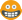but on the other hand I'm glad it's not something simple because I was racking my brain trying to figure this out!0

Frankn

### Re: Is there a ceiling function like in excel?

Well, I put a few comments in it to help explain it, but not much. Here's a verbal rundown of it

I've defined a method that accepts your float number, and the 2 numbers of the fraction you want to use for tolerance. 1/64 for example, gets written as 1.0 and 64.0 So if your number to round is 0.17578125, then you do this to round it:

my_num = 0.17578125
rounded_num = precision(my_num, 1.0, 64.0)

And rounded_num gets the returned rounded value.

The method does a few simple steps. First it takes your float and makes an integer version of your float and subtracts them. This is doing 23.123321 - 23 = 0.123321 Its just a way to isolate the decimal, which is all we want for comparison. Then it runs a loop, testing to see if the decimal is greater than 1/64. If its greater, then we loop again, adding 1 to the original 1, making 2. It tests if the decimal is greater than 2/64. If it is, it tries again, adding 1 to 2, making 3. It does this until it reaches a case where the decimal is smaller than the fraction. That tells us that our decimal lies between the most recent tested fraction, and the one just smaller than it. So your example of 0.17578125 lies between the fraction of 11/64 and 12/64.

Then we have to figure out to which it is closer. So I get a value for 11/64 and 12/64. Then subtract our decimal from both, return the absolute value and compare them. If it is closer to the 12/64 then it returns that. If its closer to 11/64, then it returns that.

That is sort of the convoluted answer.
0
Lately you've been tan, suspicious for the winter.
All my Plugins I've writtenChris Fullmer
SketchUp Team### Re: Is there a ceiling function like in excel?

Wow! Thanks for taking the time to explain it in a Ruby's for Dummies term!No way I would of come up with a solution to that problem!

That now makes total sense... now I'm off to try and implement this in my script... should funThanks again!
0

Frankn

### Re: Is there a ceiling function like in excel?

my_num = 0.17578125
frac = 16.0 # 1/16th (radix, specifically)
rounded_num = (my_num * frac).round / frac
0
Hi

Jim
Global Moderator

### Re: Is there a ceiling function like in excel?

That can't be right, you didn't even have to make any special methods to do that0
Lately you've been tan, suspicious for the winter.
All my Plugins I've writtenChris Fullmer
SketchUp Team### Re: Is there a ceiling function like in excel?

This is for measurements?

One limitation within Sketchup, is that the precision is limited to 0.001", no matter what the model units are set to.

So using a fractional float of 0.1875" may get rounded by Sketchup. Will it be 0.187" or 0.188" ??
I would suggest that you decide what it will be in your 'rounding' method...
0
I'm not here much anymore. But a PM will fire email notifications.Dan Rathbun
PluginStore Author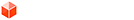### Re: Is there a ceiling function like in excel?

@Chris -Been there - just passing it along.
0
Hi

Jim
Global Moderator

### Re: Is there a ceiling function like in excel?

Here's Jim's clever solution made into a method...
Code: Select all
`def roundtofraction(num, frac)  num = num.to_f  frac = frac.to_f  return (num * frac).round / fracend`
Usage:
my_num = 0.17578125
my_frac = 16
my_num=roundtofraction(my_num, my_frac)
>>> 0.1875
0
TIGTIG
Global Moderator

### Re: Is there a ceiling function like in excel?

Jim wrote:my_num = 0.17578125
frac = 16.0 # 1/16th (radix, specifically)
rounded_num = (my_num * frac).round / frac

Dude you're a genius! That is awesome!Thank you!!
0

Frankn

### Re: Is there a ceiling function like in excel?

Chris Fullmer wrote:That can't be right, you didn't even have to make any special methods to do thatChris don't feel bad even after doing this thing called 'programming' for the last few weeks I'm still getting the hang of methods, classes and all that jazz!!0

Frankn

### Re: Is there a ceiling function like in excel?

Dan Rathbun wrote:This is for measurements?

One limitation within Sketchup, is that the precision is limited to 0.001", no matter what the model units are set to.

So using a fractional float of 0.1875" may get rounded by Sketchup. Will it be 0.187" or 0.188" ??
I would suggest that you decide what it will be in your 'rounding' method...

Yes Dan, this is for measurements.

I didn't know that limitation about Sketchup, interesting. But I'm not building a plane here just cabinets, vanties and that kind of thing, but I just don't like seeing that ~ and this script programming is addictive! You just keep adding on features and stuff you can do, I think I might have a problem.Thanks for the info
0

Frankn

### Re: Is there a ceiling function like in excel?

TIG wrote:Here's Jim's clever solution made into a method...
Code: Select all
`def roundtofraction(num, frac)  num = num.to_f  frac = frac.to_f  return (num * frac).round / fracend`
Usage:
my_num = 0.17578125
my_frac = 16
my_num=roundtofraction(my_num, my_frac)
>>> 0.1875

Thanks yet again TIG!0

Frankn

### Re: Is there a ceiling function like in excel?

Yeah TIG, thanks for wrapping it into its own method, now it feels at least slightly over-engineered0
Lately you've been tan, suspicious for the winter.
All my Plugins I've writtenChris Fullmer
SketchUp Team### Re: Is there a ceiling function like in excel?

Anything to prolong the embarrassment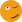0
TIGTIG
Global Moderator

### Re: Is there a ceiling function like in excel?

TIG wrote:Anything to prolong the embarrassment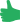0
Lately you've been tan, suspicious for the winter.
All my Plugins I've writtenChris Fullmer
SketchUp Team### Re: Is there a ceiling function like in excel?

Just a note to remind all..

... that now with Ruby in the 1.8.6 branch, we have both a ceil() and a floor() method defined for the Float class.
0
I'm not here much anymore. But a PM will fire email notifications.Dan Rathbun
PluginStore Author